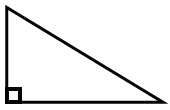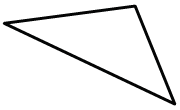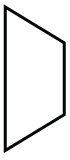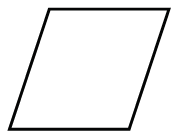Home > CC2MN > Chapter cc24 > Lesson cc24.3.1 > Problem4-82

4-82.

For each shape drawn below, choose one of the names on the list above them that best describes that shape. Be as specific as you can. If you do not remember what one of the shape names means, you may look in the glossary of this book for more information. Homework Help ✎

 right triangle scalene triangle obtuse triangle equilateral triangle parallelogram rectangle rhombus trapezoid acute triangle
1.This triangle has acute angles, so you might think it is an acute triangle. However, it is possible to be more specific, since there is a right angle.

Right or scalene triangle; there is a right angle and all of the sides are different lengths.

1.Is there an angle greater than $90°$? If so, it could be called an obtuse triangle.

1.Certainly this is a quadrilateral, but can you be more specific?

Trapezoid; there are two parallel sides with two non-parallel sides.

1.There are two pairs of parallel sides, and all sides are equal length, meaning you can be more specific than ''parallelogram.''